# Logarithm + exponential equation - math problems

#### Number of problems found: 38

• Exponential equationIn the set R solve the equation: ?
• Exponential equationSolve exponential equation (in real numbers): 98x-2=9
• Exponential equationFind x, if 625 ^ x = 5 The equation is exponential because the unknown is in the exponential power of 625
• Exponential equationSolve for x: (4^x):0,5=2/64.
• Logif ?, what is b?
• Exp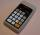If ?, then n is:
• SequenceCalculate what member of the sequence specified by ? has value 86.
• Coordinate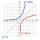Determine missing coordinate of the point M [x, 120] of the graph of the function f bv rule: y = 5x
• Compound interestCalculate time when deposit in the bank with interest 2.5% p.a. doubles.
• Car valueThe car loses value 15% every year. Determine a time (in years) when the price will be halved.
• Geometric sequenceIn the geometric sequence is a4 = 20 a9 = -160. Calculate the first member a1 and quotient q.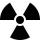After 548 hours decreases the activity of a radioactive substance to 1/9 of the initial value. What is the half-life of the substance?
• What isWhat is the annual percentage increase in the city when the population has tripled in 20 years?
• Geometric progressionIn geometric progression, a1 = 7, q = 5. Find the condition for n to sum first n members is: sn≤217.
• DemographicsThe population grew in the city in 10 years from 30000 to 34000. What is the average annual percentage increase of population?
• DecibelBy what percentage does the sound intensity increase if the sound intensity level increases by 1 dB?
• The city 3The city has 22,000 residents. How long it is expected to have 25,000 residents if the average annual population growth is 1.4%?
• Powers 3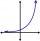2 to the power of n divided by 4 to the power of -3 equal 4. What is the vaule of n?
• Water lilies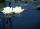Water lilies are growing on the pond and their number is doubled every day. The whole layer is covered in 12 days. How many days will it cover 8 layers?
• Geometric sequence 3In geometric sequence is a8 = 312500; a11= 39062500; sn=1953124. Calculate the first item a1, quotient q and n - number of members by their sum s_n.

Do you have an interesting mathematical word problem that you can't solve it? Submit a math problem, and we can try to solve it.

We will send a solution to your e-mail address. Solved examples are also published here. Please enter the e-mail correctly and check whether you don't have a full mailbox.

Please do not submit problems from current active competitions such as Mathematical Olympiad, correspondence seminars etc...

Logarithm - math word problems. Exponential equation - math word problems.